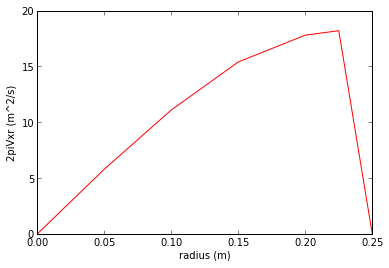# Chapter 5,Flow Measurement by Differential Head¶

## Example 5.1,Page 115¶

In :
#Variable Iniialisation
import math
rho_m =840; # kg /m^3
g =9.8; #m/ s ^2
H =0.03; #m
rho =1.2; #kg //m^3

#calculation
dp= rho_m *g*H;
v1= math.sqrt (2* dp/rho);

#result
print" Velocity(m/s) =",round(v1,2)

 Velocity(m/s) = 20.2879274447


## Example 5.2,Page 117¶

In :
%matplotlib inline
#variable initialisation
from math import pi
import numpy as np
from pylab import *
r =[0, 0.05, 0.10, 0.15, 0.20, 0.225, 0.25];
v =[19, 18.6, 17.7, 16.3, 14.2, 12.9, 0];

# We define a new variable dQ=v 2*pi*r . Accordingto the given values of r , v , we get dQ as follows
dQ =[0, 5.8, 11.1, 15.4, 17.8, 18.2, 0];

#calculation
figure()
plot (r,dQ,'r')
xlabel('radius (m)')
ylabel('2piVxr (m^2/s)')
show()
# From the graph area under the cur ve comes out to be 2 . 7 4
Q =2.74; #m^3/ s
d =0.5; # m
v =4* Q/pi /d**2;

#result
print " Rate of flow (m^3/s) =",round(Q,2);
print "Average velocity(m/s) =",round(v,2);

Welcome to pylab, a matplotlib-based Python environment [backend: module://IPython.zmq.pylab.backend_inline].
For more information, type 'help(pylab)'.Rate of flow (m^3/s) = 2.74
Average velocity(m/s) = 13.95


## Example 5.3,Page 117¶

In :
#variable declaration
from math import pi
d1 =0.1; #m
rho_Hg =13600; #kg /m^3
rho =1000; #kg /m^3
g =9.81; #m/ s ^2
H =0.8; #m
Cd =0.96;
Q =0.025; #m^3/ s

#calculation
a= pi *d1**2/4;
dp =( rho_Hg - rho )*g*H;
B =((2* dp /( rho *((Q/Cd/a)**2))) +1) **(0.25) ;
d2=d1/B;
# The shortest possible overall length of venturi is therefore an entrance cone of 7.1 cm length( 20 degrees) , a throat of 2.5 cm(0.25pipe diameter s ) and an e x i t cone o f 1 9 . 7 cm ( 7 . 5
#degrees) giving an overall length of 29.3 cm.
L =29.3; #cm

#result
print"Throat diameter (m)=",round(d2,3);
print" Overall Length(m) =",round(L,2)

Throat diameter (m)= 0.048
Overall Length(m) = 29.3


## Example 5.4,Page 119¶

In :
#Variable Decleration
Cd_o =0.65;
d =0.05;
d_o =0.025;
Cd_v =0.95;
d_v =0.038;

#calculation
# (Q o /Cd o ) ^ 2  ( ( d/ d o ) ^4 -1)=(Q v/Cd v ) ^ 2  ( ( d/ d v)^4 - 1)
#Q v=4Q o
# Q = Q v + Q o
# Q = 5Qv
Q1 =20;
Q2 =100 - Q1;
print"Flow through orifice(%)=",round(Q1)
print"Flow through venturi(%)=",round(Q2)

print"Thus 20 % of the flow passes through the orifice meter while 80% of the flow passes through the venturi."

Flow through orifice(%)= 20.0
Flow through venturi(%)= 80.0
Thus 20 % of the flow passes through the orifice meter while 80% of the flow passes through the venturi.


## Example 5.5,Page 121¶

In :
#Variable declaration
from math import pi
import math
Qa =0.003/60; # m^3/ s
Ca =20; # g / l
Co =0.126; # g / l
dp =3700; #N/m^2
p =1000; # N/m^2
d =0.1; # m
B =10/6;

#calculation
a= pi *d **2/4;
Qi=Qa *(( Ca -Co)/Co);
Q=Qi+Qa;
c= math.sqrt(2*dp/p/(1.65**4 -1**4))
Cd=Q/a/ c;

#results
print" Coefficient of discharge =",round(Cd,3)

 Coefficient of discharge = 0.967


## Example 5.6,Page 126¶

In :
#variable declaration
from math import pi
rho =850; # kg /m^3
Q =0.056; # m^3/ s
Cd =0.98;
d1 =0.2; # m
d2 =0.1; # m
g =9.81; # m/ s ^2
dz =0.3; # m

#calculation
a= pi *( d1) **2/4;
dp=rho /2*(( Q/Cd/a) **2*(( d1/d2)**4 - 1) + 2*g*( dz));

#result
print"The differential pressure (N/m^2)=",round(dp,3)

The differential pressure (N/m^2)= 23592.898


## Example 5.7,Page 128¶

In :
#variable declaration
from math import pi
import math
g =9.81; # m/ s ^2
H =0.5; #m
rho_m =1075; #kg /m^3
rho =860; #kg /m^3
B =0.225/0.075;
Cd =0.659;

#calculation
a1=pi /4*(0.225) **2;
v_t =math.sqrt(2*g*H*( rho_m - rho )/(rho )/(3**4 -1));
Q=Cd*a1* v_t ;

#result
print"Rate of flow(m^3/s) =",round(Q,5)

Rate of flow(m^3/s) = 0.00459


## Example 5.8,Page 130¶

In :
#variable decleration
from math import pi
import math
m_f =0.03; # kg
rho_f =5100; # kg /m^3
d_l =0.3; # m
d_b =0.22; # m
H_tube =0.2; # m
Cd =0.6;
H =0.1; # m
g =9.81; # m/ s ^2
rho =1000; # kg /m^3

#calculation
V_f = m_f / rho_f ;
theta =2* math.atan (( d_l - d_b )/2/ H_tube );
m=Cd*H* math.tan ( theta /2)* math.sqrt (8* V_f*g* rho *( rho_f - rho )* pi);

#result
print"Mass flow rate(kg/s) =",round(m/10,4)

Mass flow rate(kg/s) = 0.0925


## Example 5.9,Page 130¶

In :
#variable decleration
from math import pi
import math
d1 =0.05; # m
d2 =0.025; # m
Cd =0.97;
dp =1200; # N/m^2
rho =1000; #kg /m^3
H =0.15; # m
theta =2; # d e g r e e s
V_f =10**( -4) ;# m^3
g =9.81; # m/ s ^2
rho_f =8000; # kg /m^3

#calculation
B=d1/d2;
a= pi /4* d1 **2;
Q=Cd*a*  (2* dp/ (rho *(B**4 -1)))**(0.5);
Cd=Q/(H/ rho * math.tan( theta*pi/180 /2)* math.sqrt (8* V_f*g* rho *( rho_f -rho )*pi))

#result
print"Flow rate of water(m^3/s) =",round(Q,5)
print" Coeff. of discharge of the rotameter =",round(Cd,3)

Flow rate of water(m^3/s) = 0.00076
Coeff. of discharge of the rotameter = 0.7